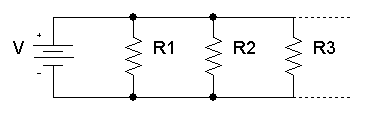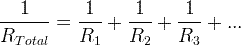# What is meant by Electrical Resistance (ohm)

## What is meant by Electrical Resistance?

Resistance is a measure of the opposition to current flow in an electrical circuit. Resistance is measured in ohms, symbolized by the Greek letter omega (Ω). Ohms are named after Georg Simon Ohm (1784-1854), a German physicist who studied the relationship between voltage, current and resistance.

If we make an analogy to water flow in pipes, the resistance is bigger when the pipe is thinner, so the water flow is decreased.

## Resistance calculation

The resistance of a conductor is resistivity of the conductor’s material times the conductor’s length divided by the conductor’s cross sectional area.R is the resistance in ohms (Ω).

ρ is the resistivity in ohms-meter (Ω×m)

l is the length of the conductor in meter (m)

A is the cross sectional area of the conductor in square meters (m2)

It is easy to understand this formula with water pipes analogy:

• when the pipe is longer, the length is bigger and the resistance will increase.
• when the pipe is wider, the cross sectional area is bigger and the resistance will decrease.

### Resistance calculation with ohm’s lawR is the resistance of the resistor in ohms (Ω).

V is the voltage drop on the resistor in volts (V).

I is the current of the resistor in amperes (A).

### Temperature effects of resistance

The resistance of a resistor increases when temperature of the resistor increases.

R2 = R× ( 1 + α(T T1) )

R2 is the resistance at temperature T2 in ohms (Ω).

R1 is the resistance at temperature T1 in ohms (Ω).

α is the temperature coefficient.

### Resistance of resistors in seriesThe total equivalent resistance of resistors in series is the sum of the resistance values:

RTotal = R1R2R3+…

### Resistance of resistors in parallelThe total equivalent resistance of resistors in parallel is given by:Electrical Voltage

Electrical Current

Kirchoff Law

Resistance

Ohm’s Law

Ampere

Difference between Voltage and Current

## 7 Feedbacks on “What is meant by Electrical Resistance (ohm)”

1.Streaming Jav Online Free - JavPlay says:

Wow, wonderful blog format! How long have you been running a blog for?
you made blogging look easy. The total look of your site is excellent,
let alone the content!

2.카지노 says:

Great blog you have here.. It’s hard to find high quality
writing like yours nowadays. I seriously appreciate people like you!
Take care!!

3.porn says:

Hello everyone, it’s my first pay a quick visit at
this website, and piece of writing is in fact fruitful in favor of me, keep up posting these types
of content.

4.xvideos says:

Saved as a favorite, I love your blog!

5.mobile legend m1 team says:

It’s very straightforward to find out any matter on web as compared to books, as I
found this post at this website.

6.google says:

Awesome article.

7.hongphong blog says:

I like the valuable information you provide in your articles.
I will bookmark your weblog and check once more here frequently.

I am relatively certain I’ll be told many new stuff right here!
Good luck for the next!